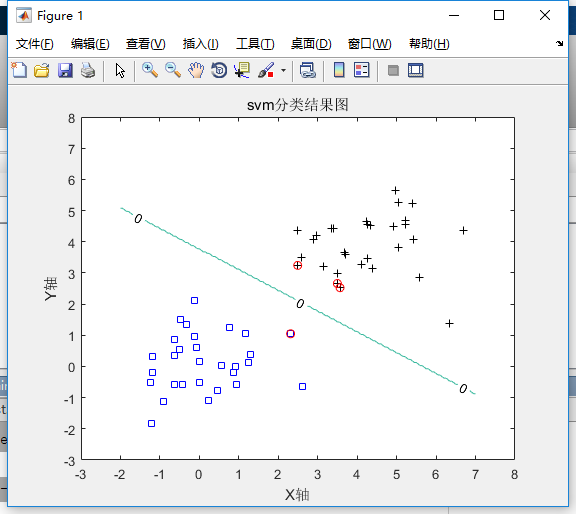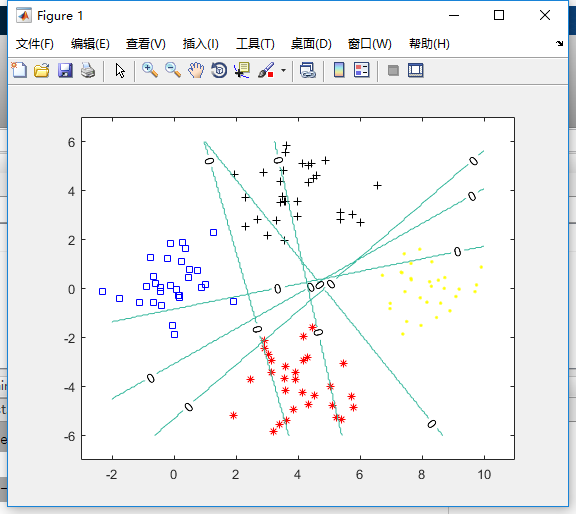• 内含libsvm工具箱、SVMs的示例程序（含代码和实例数据）、SVR的示例程序（含代码和实例数据）
• svm多分类 matlab程序

热门讨论 2014-08-22 16:23:03
采用matlab自带的svmtrain和svmpredict实现分类。
•支持向量机
• Matlab 实现线性svm两类和多类分类器

万次阅读 多人点赞 2018-01-18 21:59:43
网上有很写的好的博客讲解线性分类和SVM，本人讲解能力差，就给个链接。 http://blog.csdn.net/mm_bit/article/details/46988925 SVM实现代码 训练svm分类器实际上是解二次规划问题，matlab里用到的是...

线性分类和SVM原理

网上有很多写的好的博客讲解线性分类和SVM，本人讲解能力差，就给个链接。
http://blog.csdn.net/mm_bit/article/details/46988925

SVM实现代码

或者不想读英文文档的人可以看别人写的博客：
http://blog.csdn.net/jbb0523/article/details/50598641
svmTrain.m

function [ svm ] = svmTrain( trainData,trainLabel,kertype,C )
options=optimset;
options.LargerScale='off';
options.Display='off';

n=length(trainLabel);
H=(trainLabel'*trainLabel).*kernel(trainData,trainData,kertype);
f=-ones(n,1);
A=[];
b=[];
Aeq=trainLabel;
beq=0;
lb=zeros(n,1);
ub=C*ones(n,1);
a0=zeros(n,1);
epsilon=1e-8;
sv_label=find(abs(a)>epsilon);
svm.a=a(sv_label);
svm.Xsv=trainData(:,sv_label);
svm.Ysv=trainLabel(sv_label);
svm.svnum=length(sv_label);
end

kernel.m(更新）

function K = kernel( X,Y,type )
switch type
case 'linear'
K=X'*Y;
case 'rbf'
delta=5;
delta=delta*delta;
XX=sum(X'.*X',2);
YY=sum(Y'.*Y',2);
XY=X'.*Y;
K=abs(repmat(XX,[1 size(YY,1)])+repmat(YY',[size(XX,1) 1])-2*XY);
K=exp(-K./delta);
end
end

svmTest.m:

function result = svmTest(svm, Xt, Yt, kertype)
temp = (svm.a'.*svm.Ysv)*kernel(svm.Xsv,svm.Xsv,kertype);
%total_b = svm.Ysv-temp;
b = mean(svm.Ysv-temp);  %b取均值
w = (svm.a'.*svm.Ysv)*kernel(svm.Xsv,Xt,kertype);
result.score = w + b;
Y = sign(w+b);  %f(x)
result.Y = Y;
result.accuracy = size(find(Y==Yt))/size(Yt);
end

test.m

%------------主函数----------------
clc;
clear;
C = 10;  %成本约束参数
kertype = 'linear';  %线性核

%①------数据准备
n = 30;
%randn('state',6);   %指定状态，一般可以不用
x1 = randn(2,n);    %2行N列矩阵，元素服从正态分布
y1 = ones(1,n);       %1*N个1
x2 = 4+randn(2,n);   %2*N矩阵，元素服从正态分布且均值为5，测试高斯核可x2 = 3+randn(2,n);
y2 = -ones(1,n);      %1*N个-1

figure;  %创建一个用来显示图形输出的一个窗口对象
plot(x1(1,:),x1(2,:),'bs',x2(1,:),x2(2,:),'k+');  %画图，两堆点
axis([-3 8 -3 8]);  %设置坐标轴范围
hold on;    %在同一个figure中画几幅图时，用此句

%②-------------训练样本
X = [x1,x2];        %训练样本2*n矩阵，n为样本个数，d为特征向量个数
Y = [y1,y2];        %训练目标1*n矩阵，n为样本个数，值为+1或-1
svm = svmTrain(X,Y,kertype,C);  %训练样本
plot(svm.Xsv(1,:),svm.Xsv(2,:),'ro');   %把支持向量标出来

%③-------------测试
[x1,x2] = meshgrid(-2:0.05:7,-2:0.05:7);  %x1和x2都是181*181的矩阵
[rows,cols] = size(x1);
nt = rows*cols;
Xt = [reshape(x1,1,nt);reshape(x2,1,nt)];
%前半句reshape(x1,1,nt)是将x1转成1*（181*181）的矩阵，所以xt是2*（181*181）的矩阵
%reshape函数重新调整矩阵的行、列、维数
Yt = ones(1,nt);

result = svmTest(svm, Xt, Yt, kertype);

%④--------------画曲线的等高线图
Yd = reshape(result.Y,rows,cols);
contour(x1,x2,Yd,[0,0],'ShowText','on');%画等高线
title('svm分类结果图');
x1=xlabel('X轴');
x2=ylabel('Y轴');

分类结果：多类分类器

多类线性问题的一种解法是将多类分为多个两类分类器。
如训练集有1，2，3，4个类，让1与2作两类分类器训练得到12分类器，1与3得到13分类器，等等两两训练，得到（4-1）*4/2 6个分类器，再将测试数据用这6个分类器一一测试，如果对12，13，14三个都是正的，则该类属于1类。
代码：
multiLiner:

clc;
clear;
C=10;
kertype='linear';
%生成测试数据
n=30;
x1=randn(2,n);
x2=4+randn(2,n);

x3=randn(2,n);
x3=[x3(1,:)+4;x3(2,:)-4];

x4=randn(2,n);
x4=[x4(1,:)+8;x4(2,:)];
%可视化生成数据
plot(x1(1,:),x1(2,:),'bs',x2(1,:),x2(2,:),'k+');
hold on;
plot(x3(1,:),x3(2,:),'r*',x4(1,:),x4(2,:),'y.');
axis([-3 11 -7 7]);
hold on;
%两两合成一个训练组训练模型
trainData12=[x1,x2];
trainData13=[x1,x3];
trainData14=[x1,x4];
trainData23=[x2,x3];
trainData24=[x2,x4];
trainData34=[x3,x4];
trainLabel=[ones(1,n),-ones(1,n)];

svm_12=svmTrain(trainData12,trainLabel,kertype,C);
svm_13=svmTrain(trainData13,trainLabel,kertype,C);
svm_14=svmTrain(trainData14,trainLabel,kertype,C);
svm_23=svmTrain(trainData23,trainLabel,kertype,C);
svm_24=svmTrain(trainData24,trainLabel,kertype,C);
svm_34=svmTrain(trainData34,trainLabel,kertype,C);

%生成测试数据
[x1,x2] = meshgrid(-2:0.05:10,-6:0.05:6);  %x1和x2都是181*181的矩阵
[rows,cols] = size(x1);
nt = rows*cols;
Xt = [reshape(x1,1,nt);reshape(x2,1,nt)];
%前半句reshape(x1,1,nt)是将x1转成1*（181*181）的矩阵，所以xt是2*（181*181）的矩阵
%reshape函数重新调整矩阵的行、列、维数
Yt = ones(1,nt);
result12=svmTest(svm_12,Xt,Yt,kertype);
Yd = reshape(result12.Y,rows,cols);
contour(x1,x2,Yd,[0,0],'ShowText','on');%画等高线
hold on;
result13=svmTest(svm_13,Xt,Yt,kertype);
Yd = reshape(result13.Y,rows,cols);
contour(x1,x2,Yd,[0,0],'ShowText','on');%画等高线
hold on;
result14=svmTest(svm_14,Xt,Yt,kertype);
Yd = reshape(result14.Y,rows,cols);
contour(x1,x2,Yd,[0,0],'ShowText','on');%画等高线
hold on;
result23=svmTest(svm_23,Xt,Yt,kertype);
Yd = reshape(result23.Y,rows,cols);
contour(x1,x2,Yd,[0,0],'ShowText','on');%画等高线
hold on;
result24=svmTest(svm_24,Xt,Yt,kertype);
Yd = reshape(result24.Y,rows,cols);
contour(x1,x2,Yd,[0,0],'ShowText','on');%画等高线
hold on;
result34=svmTest(svm_34,Xt,Yt,kertype);
Yd = reshape(result34.Y,rows,cols);
contour(x1,x2,Yd,[0,0],'ShowText','on');%画等高线

%测试一个样本点属于哪一类
Xt=[10;2];
Yt=1;
result12=svmTest(svm_12,Xt,Yt,kertype);
result13=svmTest(svm_13,Xt,Yt,kertype);
result14=svmTest(svm_14,Xt,Yt,kertype);
result23=svmTest(svm_23,Xt,Yt,kertype);
result24=svmTest(svm_24,Xt,Yt,kertype);
result34=svmTest(svm_34,Xt,Yt,kertype);
if result12.Y==1&&result13.Y==1&&result14.Y==1
testLabel=1;
elseif result12.Y==-1&&result23.Y==1&&result24.Y==1
testLabel=2;
elseif result13.Y==-1&&result23.Y==-1&&result34.Y==1
testLabel=3;
elseif result14.Y==-1&&result24.Y==-1&&result34.Y==-1
testLabel=4;
else
testLabel=-1;
disp('测试点不属于这4类中');
end

分类结果：展开全文svm
• 对于组合二元支持向量机模型的多类学习，使用纠错输出码（ECOC，error-correcting output codes ）。有关详细信息，请参阅fitcecoc。 ECOC 可以用来将 Multiclass Learning 问题转化为 Binary Classification 问题。...

对于组合二元支持向量机模型的多类学习，使用纠错输出码（ECOC，error-correcting output codes ）。有关详细信息，请参阅fitcecoc。 ECOC 可以用来将 Multiclass Learning 问题转化为 Binary Classification 问题。

以下内容基于MATLAB官网的介绍文档，进行了一点个人的理解和整理，不算原创，但也不是单纯的翻译。一年的博客写作生涯，随着博士生涯进入正轨，我写博客的动力也变了，原来是纯粹为了分享和成长，想写的方法就学一学写一写。现在不行了，搞科研的方法太多了，能让人皓首穷经，慢慢地，我就变成了没有需求就没有学习的的动力。用不到的方法就不想去学了，python的学习就是这样放下的。SVM也是，这里就开个头吧，不知道以后还有没有机会深入学习，拿出来哪怕一个月的时间，从头到尾地学习。现在这篇博客里的内容缺斤少两的，想看具体的英文内容请点击链接fitcecoc

纠错输出码（ECOC）相关网页

https://www.deeplearn.me/587.html
https://blog.csdn.net/u010945683/article/details/52743515

官网的例子

Train Multiclass Model Using SVM Learners

使用支持向量机（SVM）二进制学习器训练多类纠错输出代码（ECOC）模型。

% 导入数据，Y中含有三类标签
X = meas;
Y = species;

% 使用默认选项训练多类ECOC模型。
% Mdl是ECOC多分类模型，默认情况下，fitcecoc使用SVM二进制学习器和一对一编码设计，可以使用点表示法访问Mdl属性。
Mdl = fitcecoc(X,Y)

% 显示类名称和编码设计矩阵。
% 三个类的一对一编码设计产生三个二进制学习器。CodingMat的列对应于学习器，行对应于类。
% 类顺序与Mdl.ClassNames类中的顺序相同
% 例如，CodingMat(,1)[1;1;0]，表示matlab使用分类为"setosa""versicolor"的所有观测值训练第一个支持向量机二进制学习者。
% 因为'setosa'对应于1，所以它是正类；'versicolor'对应于-1，所以它是负类。
Mdl.ClassNames
CodingMat = Mdl.CodingMatrix

% 使用单元格索引和点表示法访问每个二进制学习器。
Mdl.BinaryLearners{1}   % The first binary learner

% 计算替换类别错误。 训练数据的分类误差很小，但是分类器可能是过拟合模型。 可以使用crossval对分类器进行交叉验证，并计算交叉验证分类错误。
error = resubLoss(Mdl)

Train Multiclass Linear Classification Model

训练由多个二进制，线性分类模型组成的ECOC模型。

% 导入数据
% X是预测数据的稀疏矩阵，Y是类标签的分类向量。数据中有两个以上的类。
% 创建默认的线性分类模型模板。
t = templateLinear();
% 训练由多个二进制，线性分类模型组成的ECOC模型，这些模型可以根据文档网页上单词的频率分布来识别产品。
% 为了缩短训练时间，请转置预测数据，并指定观察值对应于列。
X = X';
rng(1); % For reproducibility
Mdl = fitcecoc(X,Y,'Learners',t,'ObservationsIn','columns')

Cross-Validate ECOC Classifier

使用SVM二进制学习器对ECOC分类器进行交叉验证，并估计分类错误。

% 交叉验证ECOC分类器
% 加载Fisher的虹膜数据集，指定预测变量数据X和标签Y。
X = meas;
Y = species;
rng(1); % For reproducibility
% 创建一个SVM模板，并标准化预测变量。
% t是一个SVM模板。 大多数模板对象属性为空，训练时，属性为其默认值。
t = templateSVM('Standardize',true)
% 训练ECOC分类器Mdl，并指定类别顺序。
Mdl = fitcecoc(X,Y,'Learners',t,...
'ClassNames',{'setosa','versicolor','virginica'});
% 使用10倍交叉验证对Mdl进行交叉验证
CVMdl = crossval(Mdl);
% 估计广义分类误差，误差越小说明分类器的泛化效果越好
genError = kfoldLoss(CVMdl)

Estimate Posterior Probabilities Using ECOC Classifier

使用SVM二进制学习器训练ECOC分类器。首先预测训练样本标签和类后验概率，然后预测网格中每个点的最大类后验概率，并可视化结果。（啊不想看了）

Speed Up Training ECOC Classifiers Using Binning and Parallel Computing

使用合并和并行计算加快训练ECOC分类器的速度。
使用具有替代分割的决策树GentleBoost集成训练一个一对多ECOC分类器。 为了加快训练速度，合并（bin应该是这个意思）数值预测器并使用并行计算。Binning只有在fitcecoc使用树型学习器时才有效。训练结束后，利用10倍交叉验证估计分类误差。

Optimize ECOC Classifier

每个SVM相关函数的实例中都有一个优化的例子

Train Multiclass ECOC Model with SVMs and Tall Arrays

创建两个基于高数据训练的多类ECOC模型。其中一个模型使用线性二进制学习器，另一个模型使用核心二进制学习器。 比较两个模型的分类误差。

其他

输入输出设置的内容也有很多，需要注意的是，在这里，可以使用多种分类器进行多分类，SCM只是其中的一种。

此外，fitcecoc只支持稀疏矩阵（稀疏矩阵是个啥？）用于训练线性分类模型。对于所有其他模型，请提供完整的预测数据矩阵。

更多的内容需要进一步学习啊，学吧，学无止境，太深了。展开全文matlab SVM 多分类
• 经典SVM算法多类分类matlab程序

千次下载 热门讨论 2013-07-17 14:17:58
• svm分类器，集成了svm多分类解决方案，支持matlab、C、python等
• %% SVM 多类示例% SVM 本质上是一对一的分类。 % 这是一个如何使用% 一比一的方法。 训练集=[ 1 10;2 20;3 30;4 40;5 50;6 66;3 30;4.1 42]; 测试集=[3 34; 1 14; 2.2 25； 6.2 63]； GroupTrain=[1;1;2;2;3;3;2;2]...matlab
• SVM是常用的一种有监督的学习模型（即给你一些输入特征，告诉你这些特征的样本是属于A，再给你一些输入特征，告诉你这些特征的样本是属于B，现在再来一些数据，来判断它们是属于哪一）。 它与Kmeans的区别在于...

SVM是常用的一种有监督的学习模型（即给你一些输入特征，告诉你这些特征的样本是属于A类，再给你一些输入特征，告诉你这些特征的样本是属于B类，现在再来一些数据，来判断它们是属于哪一类）。

它与Kmeans的区别在于kmenas是无监督的学习模型，即kmeans不需要提前知道（训练），只要你把特征给我，我根据特征分就完事了.

它与Knn的区别在于knn来一个就算一次(和集合内的其余点进行比较)，而SVM相当于一开始先把前期工作做好了（训练好），然后来一个我就根据带到训练的结果中一代就完事，

所以knn如果数据量太大或者维度太高就gg，因为我要与其数据集内比较（就算一些优化策略比较次数还是很多），而SVM有一种一劳永逸的感觉

线性SVM的一般流程：

1,数据标准化处理(减去平均值./标准差)

2,划出一个平面分割出所有的特征（也就是转化为规划模型求解）

3,训练结束后将待预测数据带入.

下面为一份训练集

我想根据资产折现力和盈利能力，活性来划分两个类别。

 编号 资产折现力 盈利能力 活性 类别 1 0.9 0.34 1.53 1 2 0.88 0.23 1.67 1 3 0.92 0.28 1.43 1 4 0.89 0.14 1.24 1 5 0.78 0.35 1.8 1 6 0.81 0.26 2.01 1 7 0.72 0.18 1.75 1 8 0.93 0.22 0.99 1 9 0.82 0.26 1.4 1 10 0.78 0.26 1.34 -1 11 0.78 0.27 1.67 -1 12 0.72 0.18 1.53 -1 13 0.69 0.16 1.2 -1 14 0.63 0.15 0.88 -1 15 0.58 0.22 1.42 -1 16 0.81 0.18 1.59 -1 17 0.67 0.21 1.21 -1 18 0.65 0.16 1.37 -1
将数据存为SVM.xls,代码如下:

clc;
clear all;
for i=1:3%%%训练样本的输入数据
X(:,i)=(X0(:,i)-mean(X0(:,i)))/std(X0(:,i));%%%标准化处理
end
[m,n]=size(X);
e=ones(m,1);
D=[X0(:,4)];%%%训练样本的输出
B=zeros(m,m);
for i=1:m
B(i,i)=1;
C(i,i)=D(i,1);
end
A=[-X(:,1).*D,-X(:,2).*D,-X(:,3).*D,D,-B];
b=-e;
f=[0,0,0,0,ones(1,m)];
lb=[-inf,-inf,-inf,-inf,zeros(1,m)]';
x=linprog(f,A,b,[],[],lb);
W=[x(1,1),x(2,1),x(3,1)];
CC=x(4,1);
X1=[X(:,1),X(:,2),X(:,3)];
R1=X1*W'-CC;
R2=sign(R1);
disp('程序输出结果为:');
R=[R1,R2]
虽然有很小误差但是可以接受。

但是常见的并不是分为两类，比如葡萄酒那题分为4,5类怎么办（当然那题是无监督的，直接层次聚类或KEMANS就OK了）

假如我有三类要划分，他们是A、B、C。

于是我在抽取训练集的时候，分别抽取

（1）A所对应的向量作为正集(为1)，B，C所对应的向量作为负集(剩下的分为-1)；

（2）B所对应的向量作为正集，A，C所对应的向量作为负集；

（3）C所对应的向量作为正集，A，B所对应的向量作为负集；

使用这三个训练集分别进行训练，然后的得到三个训练结果文件。

在测试的时候，把对应的测试向量分别利用这三个训练结果文件进行测试。

最后每个测试都有一个结果f1(x),f2(x),f3(x).

于是最终的结果便是这四个值中最大的一个作为分类结果。

也就是说流程如下:

1,将数据整理成三个xls

2,对于第i个xls代表输入第i类的测试集，属于第i类的就标记结果为1,剩下的为-1.

3，每一个均划分平面

4,带入预测数据.

5,对于每一个预测数据取最大的那个xls的值作为结果，也就是说它属于第i类。

新的数据集如下:

 编号 资产折现力 盈利能力 活性 类别 1 0.9 0.34 1.53 1 2 0.88 0.23 1.67 1 3 0.92 0.28 1.43 1 4 0.89 0.14 1.24 1 5 0.78 0.35 1.8 1 6 0.81 0.26 2.01 1 7 0.72 0.18 1.75 2 8 0.93 0.22 0.99 2 9 0.82 0.26 1.4 2 10 0.78 0.26 1.34 2 11 0.78 0.27 1.67 2 12 0.72 0.18 1.53 2 13 0.69 0.16 1.2 3 14 0.63 0.15 0.88 3 15 0.58 0.22 1.42 3 16 0.81 0.18 1.59 3 17 0.67 0.21 1.21 3 18 0.65 0.16 1.37 3
将数据处理后分别存入SVM2.xls,SVM3.xls,SVM4.xls

代码如下:

clc;
clear all;
for i=1:3%%%训练样本的输入数据
X(:,i)=(X0(:,i)-mean(X0(:,i)))/std(X0(:,i));%%%标准化处理
end
[m,n]=size(X);
e=ones(m,1);
D=[X0(:,4)];%%%训练样本的输出
B=zeros(m,m);
for i=1:m
B(i,i)=1;
C(i,i)=D(i,1);
end
A=[-X(:,1).*D,-X(:,2).*D,-X(:,3).*D,D,-B];
b=-e;
f=[0,0,0,0,ones(1,m)];
lb=[-inf,-inf,-inf,-inf,zeros(1,m)]';
x=linprog(f,A,b,[],[],lb);
W=[x(1,1),x(2,1),x(3,1)];
CC=x(4,1);
X1=[X(:,1),X(:,2),X(:,3)];
R1=X1*W'-CC;
R=R1;

%%%处理表二
for i=1:3%%%训练样本的输入数据
X(:,i)=(X0(:,i)-mean(X0(:,i)))/std(X0(:,i));%%%标准化处理
end
[m,n]=size(X);
e=ones(m,1);
D=[X0(:,4)];%%%训练样本的输出
B=zeros(m,m);
for i=1:m
B(i,i)=1;
C(i,i)=D(i,1);
end
A=[-X(:,1).*D,-X(:,2).*D,-X(:,3).D,D,-B];
b=-e;
f=[0,0,0,0,ones(1,m)];
lb=[-inf,-inf,-inf,-inf,zeros(1,m)]’;
x=linprog(f,A,b,[],[],lb);
W=[x(1,1),x(2,1),x(3,1)];
CC=x(4,1);
X1=[X(:,1),X(:,2),X(:,3)];
R1=X1
W’-CC;
R=[R,R1];

%%%处理表三
for i=1:3%%%训练样本的输入数据
X(:,i)=(X0(:,i)-mean(X0(:,i)))/std(X0(:,i));%%%标准化处理
end
[m,n]=size(X);
e=ones(m,1);
D=[X0(:,4)];%%%训练样本的输出
B=zeros(m,m);
for i=1:m
B(i,i)=1;
C(i,i)=D(i,1);
end
A=[-X(:,1).*D,-X(:,2).*D,-X(:,3).D,D,-B];
b=-e;
f=[0,0,0,0,ones(1,m)];
lb=[-inf,-inf,-inf,-inf,zeros(1,m)]’;
x=linprog(f,A,b,[],[],lb);
W=[x(1,1),x(2,1),x(3,1)];
CC=x(4,1);
X1=[X(:,1),X(:,2),X(:,3)];
R1=X1
W’-CC;
R=[R,R1];
for i=1:size(R,1)
[c,d]=max(R(i,:));
t(i,1)=c;
t(i,2)=d;%%%判断属于哪一个类别
end
disp(‘输出结果为:’);
t

t =

91.4813    1.0000

105.2576    1.0000

91.4428    1.0000

5.0052    1.0000

10.0521    1.0000

105.9796    1.0000

-0.7916    2.0000

0.5677    2.0000

-0.7547    2.0000

-1.0000    3.0000

-1.2244    2.0000

-0.3183    3.0000

1.1881    3.0000

2.9200    3.0000

1.2706    3.0000

-0.0850    2.0000

1.0000    3.0000

1.1044    3.0000

结果还不错~~

展开全文SVM MATLAB 多分类
• matlab平台下实现的SVM二分类问题；共两个：一个是线性分类问题；另一个是非线性分类问题。
• matlab实现多分类svm

千次阅读 2020-04-19 00:41:08
matlab独自实现分类svm-csdn matlab独自实现分类svm-git-hub 设计思路 SVM也叫支持向量机，其是一个二分类器，但是对于分类，SVM也可以实现。主要方法就是训练个二分类器。常见的有以下两种方式： 一对...

多分类SVM

设计思路

SVM也叫支持向量机，其是一个二类分类器，但是对于多分类，SVM也可以实现。主要方法就是训练多个二类分类器。常见的有以下两种方式：

• 一对一（one-vs-one）
给定m个类，对m个类中的每两个类都训练一个分类器，总共的二类分类器个数为 m(m-1)/2 .比如有三个类，1，2，3，那么需要有三个分类器，分别是针对：1和2类，1和3类，2和3类。对于一个需要分类的数据x,它需要经过所有分类器的预测，最后使用投票的方式来决定x最终的类属性。
• 一对多（one-vs-rest）
给定m个类，需要训练m个二类分类器。其中的分类器 i 是将 i 类数据设置为类1（正类），其它所有m-1个i类以外的类共同设置为类2（负类），这样，针对每一个类都需要训练一个二类分类器，最后，我们一共有 m 个分类器。对于一个需要分类的数据 x，通常选择置信度最大的类别标记为分类结果。

由于类别较多，本实验采用一对多方式预测

代码

function y_predict = my_MultiSvm(X_train, y_train, X_test)
% multi svm
% one vs all 模型
% Input:
% X_train: n*m矩阵 n为训练集样本数 m为特征数
% y_train: n*1向量 为训练集label，支持任意多种类
% X_test: n*m矩阵 n为测试集样本数 m为特征数
% Output:
% y_predict: n*1向量 测试集的预测结果
%
%

y_labels = unique(y_train);
n_class = size(y_labels, 1);
models = cell(n_class, 1);
% 训练n个模型
for i = 1:n_class
class_i_place = find(y_train == y_labels(i));
svm_train_x = X_train(class_i_place,:);
sample_num = numel(class_i_place);
class_others = find(y_train ~= y_labels(i));
randp = randperm(numel(class_others));
svm_train_minus = randp(1:sample_num)';
svm_train_x = [svm_train_x; X_train(svm_train_minus,:)];
svm_train_y = [ones(sample_num, 1); -1*ones(sample_num, 1)];
disp(['生成模型：', num2str(i)])
models{i} = fitcsvm(svm_train_x, svm_train_y);
end
test_num = size(X_test, 1);
y_predict = zeros(test_num, 1);
% 对每条数据，n个模型分别进行预测，选择label为1且概率最大的一个作为预测类别
for i = 1:test_num
if mod(i, 100) == 0
disp(['预测个数：', num2str(i)])
end
bagging = zeros(n_class, 1);
for j = 1:n_class
model = models{j};
[label, rat] = predict(model, X_test(i,:));
bagging(j) = bagging(j) + rat(2);
end
[maxn, maxp] = max(bagging);
y_predict(i) = y_labels(maxp);
end
end

展开全文• 在当前数据挖掘和机器学习领域，最为热门的话题莫过于SVM和Boosting方法了。只要是涉及到这两个主题，那么论文就会容易被杂志和会议接受了。看来不管做什么，都讲究眼球效应啊。搞研究其实也有点类似超级女声，呵呵...
• 提供的 MATLAB 函数可用于使用基于树状图的支持向量机 (D-SVM) 对数据集进行训练和执行多类分类。 两个主要功能是： Train_DSVM：这是用于训练的函数Classify_DSVM：这是用于 D-SVM 分类的函数示例：使用fisheriris ...matlab
• SVM算法的代码，用matlab实现的，可直接用，很方便，下载即可用！该算法可用于机器学习分类研究，是一种典型的分类算法，非常适合论文实验。
• 基于MATLABsvm分类器代码实现基于MATLABsvm分类器代码实现MATLAB
• 一个简单的svm分类，未进行参数优化，请大佬们指教（凑字数）
• 文章目录SVM 分类对带标签的高光谱数据建立分类模型代码1.训练集、测试集2、数据归一化3、参数选择4、模型训练5、模型测试 SVM 分类 采用libSVM包进行分类 对带标签的高光谱数据建立分类模型 数据描述：三个...matlab svm 分类算法
• 采用函数 fitcecoc 进行SVM二分类/分类模型训练；3.采用10折交叉验证，将Mdl转化为 CVMdl，减少泛化误差4.将泛化误差ossLoss作为模型的评价指标示例1：鸢尾花数据集irisfunction [CVMdl,oosLoss]=SVM3()load ...
• MATLAB中的fisheriris分类，使用SVM算法，图像显示
• 这也接受所有选项作为 varargin 无需担心这是完美的工作我使用以下参考进行分类多类支持向量机多类 SVM 旨在通过使用支持向量机为实例分配标签，其中标签是从几个元素的有限集合中提取的。 这样做的主要方法是将...matlab
• 鸢尾花数据集，用于svm分类的数据集，MATLAB和python都可用。其中包括txt格式和data格式，只需要使用libsvm的包就可以调用。
•SVM MATLAB
• 基于matlabsvm的留一法代码实现 说明 本代码是实现论文“基于旋转模式的移动设备佩戴位置识别方法”中的实验，提供给需要的同学参考，具体的算法细节请参考该文，这里仅作实现。另外我发布的代码是留一法，原文中...
• 利用MATLAB实现SVM分类 %% LibSVM clc;clear;close all; %% 加载文件 [Iris1,Iris2,Iris3,Iris4,IrisClass]=textread('iris.data','%f%f%f%f%s','delimiter',','); Wine=load('wine.data'); % 仅读数字 Abalone=...matlab 机器学习
• MATLAB 简单实现了的SVM的算法（软间隔最大化），svm_main.m是主程序文件。 介绍博客：https://blog.csdn.net/qq_24783597/article/details/80628878SVM
• 使用libsvm 实现MNIST数据库手写数字识别，正确率98.14. 包含matlab程序，libsvm库，以及60000张训练数据10000张测试数据
• libsvm - 支持类别分类的svm工具箱（matlab）. 它扩展了matlab自带的svm分类器的功能（只能完成2分类），可配合DeepLearnToolbox使用libsvm
• 完全MATLAB代码，包涵大量注释，个子程序方便其他算法的替换，基于DSIFT+BOW+SVM的物体分类算法，只要下载caltech101，压缩包里附带下载网址，不用其他配置，方便学习。...

matlab多类svmmatlab 订阅